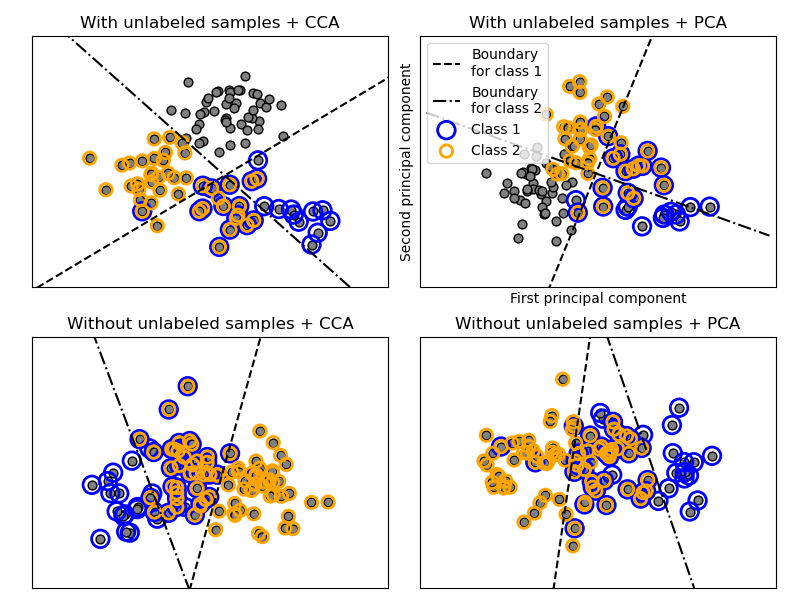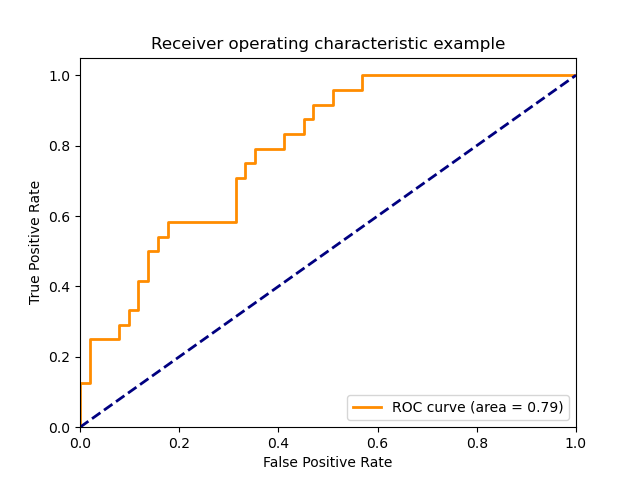# sklearn.multiclass.OneVsRestClassifier¶

class sklearn.multiclass.OneVsRestClassifier(estimator, *, n_jobs=None)

[源码]

estimator estimator object

n_jobs int or None, optional (default=None)

v0.20版中已更改：n_jobs默认从1更改为None

estimators_ list of n_classes estimators

classes_ array, shape = [n_classes]

n_classes_ int

label_binarizer_ LabelBinarizer object

multilabel_ boolean

>>> import numpy as np>>> from sklearn.multiclass import OneVsRestClassifier>>> from sklearn.svm import SVC>>> X = np.array([...     [10, 10],...     [8, 10],...     [-5, 5.5],...     [-5.4, 5.5],...     [-20, -20],...     [-15, -20]... ])>>> y = np.array([0, 0, 1, 1, 2, 2])>>> clf = OneVsRestClassifier(SVC()).fit(X, y)>>> clf.predict([[-19, -20], [9, 9], [-5, 5]])array([2, 0, 1])

decision_function(X) 返回每个样本到决策边界的每个样本的距离。
fit(X, y) 拟合基础估计量。
get_params([deep]) 获取此估计量的参数。
partial_fit(X, y[, classes]) 部分拟合基础估计量
predict(X) 使用基础估计量预测多类别目标。
predict_proba(X) 概率估计。
score(X, y[, sample_weight]) 返回给定测试数据和标签上的平均准确度。
set_params(**params) 设置此估算量的参数。
__init__(estimator, *, n_jobs=None)

[源码]

decision_function(X)

[源码]

X array-like of shape (n_samples, n_features)

T array-like of shape (n_samples, n_classes)
*在版本0.19中进行了更改：*输出形状已更改为(n_samples,)符合scikit-learn二进制分类的约定。
fit(X, y)

[源码]

X (sparse) array-like of shape (n_samples, n_features)

y (sparse) array-like of shape (n_samples,) or (n_samples, n_classes)

self
get_params(deep=True)

[源码]

deep bool, default=True

params mapping of string to any

property multilabel_

partial_fit(X, y, classes=None)

[源码]

X (sparse) array-like of shape (n_samples, n_features)

y (sparse) array-like of shape (n_samples,) or (n_samples, n_classes)

classes array, shape (n_classes, )

self
predict(X)

[源码]

X (sparse) array-like of shape (n_samples, n_features)

y (sparse) array-like of shape (n_samples,) or (n_samples, n_classes)

predict_proba(X)

[源码]

X array-like of shape (n_samples, n_features)

T (sparse) array-like of shape (n_samples, n_classes)

score(X, y, sample_weight=None)

[源码]

X array-like of shape (n_samples, n_features)

y array-like of shape (n_samples,) or (n_samples, n_outputs)
X的真实标签。
sample_weight array-like of shape (n_samples,), default=None

score float
self.predict(X) wrt. y.的平均准确度。
set_params(**params)

[源码]

**params dict

self object

## sklearn.multiclass.OneVsRestClassifier使用实例¶